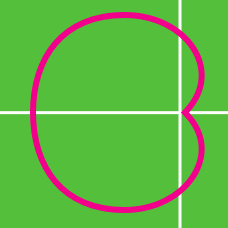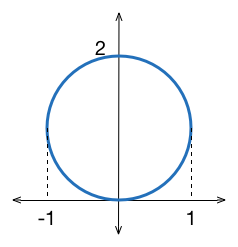Calculus

# Polar Coordinates - Problem SolvingWhat is the polar equation of the above graph?

Consider the graphs of two polar functions $r=6\sin \theta, r=1+\sin \theta.$ How many intersection points do they have?

What is the number of regions bounded by the polar curve $r=\cos 2\theta$?

If the two polar equations $r=\frac{1}{\cos \theta -\sin \theta}, r^2=181$ are converted to Cartesian coordinates, what is the sum of the $x$-coordinates of all intersection points of the two curves?

How many times do the following curves in polar coordinates intersect $r=\cos 4\theta$ and $r=\frac{1}{2}$?

×